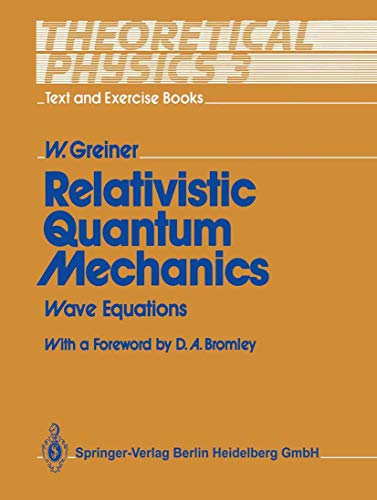# Theoretical Physics - Text and Exercise Books: Volume 3: Relativistic Quantum Mechanics. Wave Equations

## Greiner, Walter

4,5 valoración promedio
( 2 valoraciones por Goodreads )Ver todas las copias de esta edición ISBN.
Reseńa del editor:

Relativistic Quantum Mechanics - Wave Equations concentrates mainly on the wave equations for spin-0 and spin-1/2 particles. Chapter 1 deals with the Klein-Gordon equation and its properties and applications. The chapters that follow introduce the Dirac equation, investigate its covariance properties and present various approaches to obtaining solutions. Numerous applications are discussed in detail, including the two-center Dirac equation, hole theory, CPT symmetry, Klein's paradox, and relativistic symmetry principles. Chapter 15 presents the relativistic wave equations for higher spin (Proca, Rarita-Schwinger, and Bargmann-Wigner). The extensive presentation of the mathematical tools and the 62 worked examples and problems make this a unique text for an advanced quantum mechanics course.

Reseńa del editor:

The key to understanding quantum theory is to reinforce lecture attendance and textual study by working through plenty of representative and detailed examples. Firm belief in this principle led the author to develop his teaching style and to transform it into a text. This text features a large number of examples and exercises involving many of the most advanced topics in quantum theory. These examples give practical demonstrations of how to use the often subtle mathematics behind quantum theory. It presents a very important part of relativistic quantum mechanics: the wave equations for spin 0 and spin +. Chapter 1 deals with the Klein-Gordon equation and its properties and applications. The following chapters introduce the Dirac equation, investigate its covariance properties and present various approaches to obtain solutions. Numerous applications are discussed in detail, including the two-centre Dirac equation, hole theory and PCT symmetry, Klein's paradox and relativistic symmetry principles. The extensive presentation of the mathematical tools and the 62 worked examples and problems make this a useful text for the advanced quantum mechanics course.

"Sobre este título" puede pertenecer a otra edición de este libro.

Comprar nuevo Ver este artículo
EUR 92,24

Gastos de envío: GRATIS

Destinos, gastos y plazos de envío

## 1.Theoretical Physics - Text And Exercise Books: Volume 3: Relativistic Quantum Mechanics. Wave Equations

ISBN 10: 3540509860 ISBN 13: 9783540509868
Librería
GoldBooks
(Austin, TX, Estados Unidos de America)

Descripción Springer. Condición: new. Nş de ref. del artículo: think3540509860

Comprar nuevo
EUR 92,24
Convertir moneda
Gastos de envío: GRATIS
Destinos, gastos y plazos de envío

## 2.Theoretical Physics - Text and Exercise Books: Volume 3: Relativistic Quantum Mechanics. Wave Equati

ISBN 10: 3540509860 ISBN 13: 9783540509868